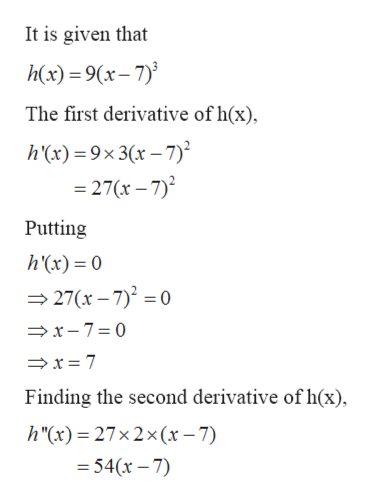# Find all relative extrema of the function. (If an answer does not exist, enter DNE.)h(x) = 9(x − 7)3relative maximum    (x, y) =        relative minimum    (x, y) =

Question
18 views

Find all relative extrema of the function. (If an answer does not exist, enter DNE.)

h(x) = 9(x − 7)3
relative maximum     (x, y)  =

relative minimum     (x, y)  =

check_circle

Step 1

Given: -

Step 2

To find: -

Step 3

Calculation...help_outlineImage TranscriptioncloseIt is given that h(x) 9(x-7 The first derivative of h(x) h(x)9x3(x-7) 27(x-7)2 Putting h'(x) 0 27(x-7)2 0 x-7 0 x 7 Finding the second derivative of h(x) h"(x) = 27x2 x (x-7) 54(x-7) fullscreen

### Want to see the full answer?

See Solution

#### Want to see this answer and more?

Solutions are written by subject experts who are available 24/7. Questions are typically answered within 1 hour.*

See Solution
*Response times may vary by subject and question.
Tagged in

### Derivative